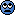# Kinematics question help

• DracoMalfoy
I'm assuming it's B. Now I'm not sure if that's what the problem want me to answer.Now that I really think about it... I see the ball traveling FROM the wall or whatever is projecting it to the racket and back in the other direction... but the problem doesn't state which is the negative... so I'm assuming it's B. Now I'm not sure if that's what the problem want me to answer.f

## Homework Statement

A ball is traveling at 3m/s then bounces off of a tennis racket and returns at 2m/s. If the ball is in contact with the racket for 12ms, what is the average acceleration?

## Homework Equations

average acceleration and conversion

a=Vf-Vi/Tf-Ti
1s= 1000ms

## The Attempt at a Solution

Before I started, I converted 12ms to s and got 0.012s. I know the equation for avg acceleration. but I feel like I'm missing a step. I plug in the numbers and get an unrealistic solution. The answer comes out negative. I feel like I am missing something. Or I am making it too complicated for myself.

Please show your actual steps. Dont just say ”I put in the numbers and think I got it wrong”. How on Earth are we to see if you got it wrong or not if you don't show us what you did?

## Homework Statement

A ball is traveling at 3m/s then bounces off of a tennis racket and returns at 2m/s. If the ball is in contact with the racket for 12ms, what is the average acceleration?

## Homework Equations

average acceleration and conversion

a=Vf-Vi/Tf-Ti
1s= 1000ms

## The Attempt at a Solution

Before I started, I converted 12ms to s and got 0.012s. I know the equation for avg acceleration. but I feel like I'm missing a step. I plug in the numbers and get an unrealistic solution. The answer comes out negative. I feel like I am missing something. Or I am making it too complicated for myself.
Everything you have showed us so far looks correct. A negative acceleration is not necessarily wrong. The magnitude of the acceleration should be pretty large to cause that change in velocity in such a short time. Why don't you show us your calculations so we can see if they are correct?

Please show your actual steps. Dont just say ”I put in the numbers and think I got it wrong”. How on Earth are we to see if you got it wrong or not if you don't show us what you did?

I subtracted 2m/s from 3m/s and then divided by the time 0.012s.

Everything you have showed us so far looks correct. A negative acceleration is not necessarily wrong. The magnitude of the acceleration should be pretty large to cause that change in velocity in such a short time. Why don't you show us your calculations so we can see if they are correct?

I subtracted 2m/s from 3m/s and then divided by the time 0.012s and got -83.3m/s^2I subtracted 2m/s from 3m/s and then divided by the time 0.012s and got -83.3m/s^2Velocity has direction. The change in speed 1m/s, but what is the change in velocity?

I subtracted 2m/s from 3m/s and then divided by the time 0.012s and got -83.3m/s^2You need to think about which direction the ball is traveling in before and after hitting it with the racket. Does the sign of the velocity change?

You need to think about which direction the ball is traveling in before and after hitting it with the racket. Does the sign of the velocity change?

I'm guessing that after it hits the racket it goes in the opposite direction so should 2m/s be negative?

I'm guessing that after it hits the racket it goes in the opposite direction so should 2m/s be negative?
Yes.

Yes.

Okay. I changed that. So i subtracted 3m/s from -2m/s and got -5. Then I divided by 0.012s and got -417m/s^2. but the question is multiple choice. The answers are:

A) 0.417m/s^2
B) -56m/s^2
C) 0m/s^2
D) 83.3m/s^2
E) 417m/s^2

Okay. I changed that. So i subtracted 3m/s from -2m/s and got -5. Then I divided by 0.012s and got -417m/s^2. but the question is multiple choice. The answers are:

A) 0.417m/s^2
B) -56m/s^2
C) 0m/s^2
D) 83.3m/s^2
E) 417m/s^2

3m/s is the positive direction. 2m/s is the negative direction I'm assuming since its returning. B is the only negative answer thoughDoes the sign depend on which direction you define as negative and which you define as positive? Is it specified in the problem which is the positive direction?

Does the sign depend on which direction you define as negative and which you define as positive? Is it specified in the problem which is the positive direction?

Now that I really think about it... I see the ball traveling FROM the wall or whatever is projecting it to the racket and back in the other direction... but the problem doesn't state which is the negative direction.

Now that I really think about it... I see the ball traveling FROM the wall or whatever is projecting it to the racket and back in the other direction... but the problem doesn't state which is the negative direction.
Then which answer can be correct knowing that the people who constructed the problem may have defined the positive direction in either direction?

Then which answer can be correct knowing that the people who constructed the problem may have defined the positive direction in either direction?

417m/s^2? E? I first thought D) 83.3m/s^2 but that was incorrect.

417m/s^2? E?
Yes.